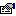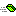## RijndaelManaged

Represents an implementation of the Rijndael symmetric block cipher algorithm

### Public:

#### Properties:

NameDescriptionBlockSize (get) Returns the block size, in bits, used by this algorithm.BlockSize (let) Sets the block size, in bits, used by this algorithm.FeedbackSize (get) Returns the Feedback Size in bits.FeedbackSize (let) Sets the Feedback size in bits.IV (get) Returns the Initialization Vector.IV (let) Sets the Initialization Vector to be used.Key (get) Returns the current secret key.Key (let) Sets the secret key.KeySize (get) Returns the key size in bits.KeySize (let) Sets the key size in bits.LegalBlockSizes (get) Returns a list of legal block sizes.LegalKeySizes (get) Returns a list of valid key sizes supported by this algorithm.Mode (get) Returns the Cipher mode this algorithm will use.Mode (let) Sets the Cipher mode this algorithm will use.Padding (get) Returns the padding mode being used.Padding (let) Sets the padding mode to be used.

#### Methods:

NameDescriptionClear Clears the Key and IV arrays.CreateDecryptor Returns a new cipher used to decrypt data.CreateEncryptor Returns a new cipher used to encrypt data.Equals This function determines if the value passed in is the same as the current object instance. Meaning, are the Value and this object the same object in memory.GenerateIV Generates a random IV array to use.GenerateKey Generates a random key to be used.GetHashCode Returns a psuedo-unique number used to help identify this object in memory. The current method is to return the value obtained from ObjPtr. If a different method needs to be impelmented then change the method here in this function.ToString Returns a string representation of this object instance. The default method simply returns the application name and class name in which this class resides.ValidKeySize Tests if a specific bit length is valid for a key.

#### Remarks

Details about Rijndael can be found here: http://www.iaik.tugraz.at/research/krypto/AES/old/~rijmen/rijndael/

#### Examples

This example shows a very simple method of encrypting then decrypting a String value.

```Private Sub Main()
Dim Key As New RijndaelManaged
Dim Buffer() As Byte
Dim PlainText As String

' Encrypt a string to a byte array.
Buffer = Encrypt("This is some plaintext!", Key)

Debug.Print "Encrypted data"
PrintBytes Buffer
Debug.Print

' Decrypt the byte array back to a string.
PlainText = Decrypt(Buffer, Key)

' Display the plaintext value to the console.
Debug.Print "Original data"
Debug.Print PlainText
End Sub

Private Sub PrintBytes(ByRef Bytes() As Byte)
Dim i As Long

For i = LBound(Bytes) To UBound(Bytes)
Debug.Print Object.ToString(Bytes(i), "X2"); " ";
Next

Debug.Print
End Sub

' Encrypt the string.
Private Function Encrypt(ByVal PlainText As String, ByVal Key As SymmetricAlgorithm) As Byte()
Dim Encryptor As ICryptoTransform
Dim Buffer() As Byte

Buffer = Encoding.UTF8.GetBytes(PlainText)
Set Encryptor = Key.CreateEncryptor
Encrypt = Encryptor.TransformFinalBlock(Buffer, 0, CorArray.Length(Buffer))
End Function

' Decrypt the byte array.
Public Function Decrypt(ByRef CypherText() As Byte, ByVal Key As SymmetricAlgorithm) As String
Dim Decryptor As ICryptoTransform
Dim Buffer() As Byte

Set Decryptor = Key.CreateDecryptor
Buffer = Decryptor.TransformFinalBlock(CypherText, 0, CorArray.Length(CypherText))
Decrypt = Encoding.UTF8.GetString(Buffer)
End Function

' This example produces the following output.
'
'    Encrypted Data (may be different because key is generated)
'    FA 5B 69 FB 30 48 B6 08 C3 68 4C 63 45 BE F9 FA 17 DC 4D B9 EC FC E7 84 3A 35 CB 41 1C 5E 1D E5
'
'    Original Data
'    This is some plaintext!
```